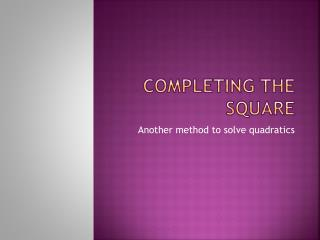Download PresentationCompleting the square

# Completing the square

Download Presentation## Completing the square

- - - - - - - - - - - - - - - - - - - - - - - - - - - E N D - - - - - - - - - - - - - - - - - - - - - - - - - - -
##### Presentation Transcript

1. Completing the square Another method to solve quadratics

2. What does a square look like? Is this a square? What about this one? This one? Why isn’t the last picture a square?

3. Question… • What would we need to do to make this a square? • Right, we would need to fill in the missing bottom section.

4. Same with quadratics • We can model a quadratic expression like this • with tiles like this... This is the Split the x piece in 2 and put 1/2  here rest here The constant (4) goes here

5. Now it’s your turn • Each group has some squares and rectangles. Practice showing completing the square for the following problems: • Write the problems on a piece of paper and fill in the blanks when you find the number of small squares needed to complete the square. + 8x +____

6. What did you notice? • Take 3 minutes and discuss some of the things you notice about what happened when you worked out those problems. Write your observations under the problems on the paper. • What were the answers you got for the problems + 8x + 16